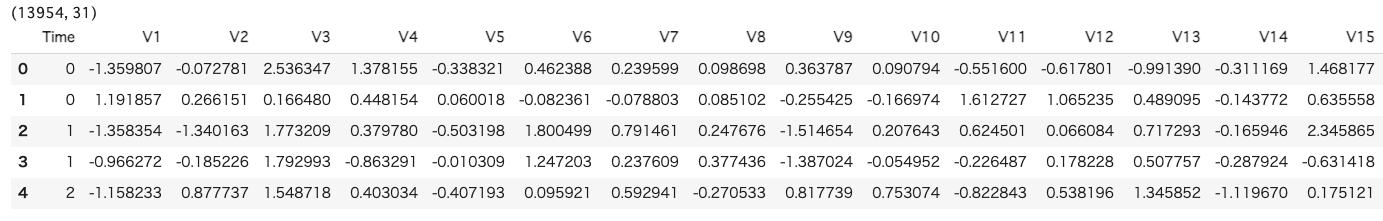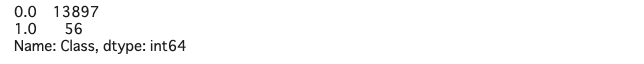# [PYTHON] How to deal with imbalanced data

• Articles sent by data scientists from the manufacturing industry
• This time, we will organize how to deal with imbalanced data.

## Introduction

When I had to carry out a classification problem in my work, I sometimes dealt with imbalanced data, so when I was investigating how to deal with it, I found methods called "under sampling" and "over sampling", so I will organize them. ..

## What is imbalanced data?

Imbalanced data refers to data that has a large bias in the distribution of objective variables. Simply put, when there is data such as label 0 being 99% and label 1 being 1%, it is known that if a classification model is generated without any special measures, the classification accuracy of the minority will be low. (It is natural that 99% of all labels are 0, so it is natural). When dealing with imbalanced data, it is often the purpose to identify minority data, so it is necessary to devise it.

## What is under sampling?

This is a method of randomly extracting from the majority data to match the number of minority data. This method is easy, but it should be noted that it causes the following two problems because it discards useful data for training at the time of sampling and the total number of data is insufficient.

1. The problem that the variance of the learned classifier becomes large
2. The problem that the posterior distribution obtained after learning is distorted

Countermeasures and research have been conducted on the above issues, but this time we will omit them.

## What is over sampling?

It is to supplement the shortage data based on the minority data. Here's a simple way to generate new data:

• Numerical data: Randomly generated using mean and variance
• Category data: Randomly generated composition ratio as weight

However, with this method, the correlation between features cannot be considered. For example, despite the correlation between height and weight, data of height 170 cm and weight 60 kg may be generated, which may affect the accuracy of the model.

SMOTE (Synthetic Minority Oversampling TEchnique) is a method to solve such problems. SMOTE is a method of connecting each data point with the smaller label with a line and randomly generating any point on the line segment as artificial data. There are some extended methods, but I will omit them this time.

## Correspondence to unbalanced data (implementation)

This time, we will use the Credit Card Fraud Detection dataset provided by kaggle. Each record has a value indicating whether or not it is abused (1 is abused), but most of them are 0, which is a very unbalanced data set.

The python code is below.

``````#Import required libraries
import pandas as pd
from sklearn.datasets import make_classification

from sklearn.ensemble import GradientBoostingClassifier
from sklearn.model_selection import train_test_split
from sklearn.metrics import accuracy_score, confusion_matrix

#Read Credit Card Fraud Detection data

print(df.shape)
``````Next, whether it is fraudulent or not is in the'Class' column, so let's look at the data bias.

``````#Check the number of data in the classification class
df['Class'].value_counts()
``````You can see that it is biased. At first, I will try to create a classification model with a gradient boosting tree without any support.

``````#Confirmation of missing values
df.isnull().sum()

#Delete missing value data
df = df.dropna()

#Separate data for training and validation
x = df.iloc[:, 1:30]
y = df['Class']
x_train, x_test, y_train, y_test = train_test_split(x, y, random_state = 40)

#Classification model creation
model.fit(x_train, y_train)

#Predicted test data with the created model
y_pred = model.predict(x_test)

#Accuracy and confusion matrix
print('Confusion matrix(test):\n{}'.format(confusion_matrix(y_test, y_pred)))
print('Accuracy(test) : %.4f' %accuracy_score(y_test, y_pred))
# Confusion matrix(test):
# [[3473    0]
#  [   2   14]]
# Accuracy(test) : 0.9994

#Precision and Recall
tn, fp, fn, tp = confusion_matrix(y_test, y_pred).ravel()
print('precision : %.4f'%(tp / (tp + fp)))
print('recall : %.4f'%(tp / (tp + fn)))
# precision : 1.0000
# recall : 0.8750

#F value
f1_score(y_pred, y_test)
# 0.9333333333333333
``````

Next, I will try Under Sampling.

``````#Library
from imblearn.under_sampling import RandomUnderSampler

#Save the number of positive examples
positive_count_train = int(y_train.sum())
print('positive count:{}'.format(positive_count_train))

#Downsampling negative examples until 10% positive
rus = RandomUnderSampler(sampling_strategy={0:positive_count_train*9, 1:positive_count_train}, random_state=40)

#Reflected in learning data
x_train_resampled, y_train_resampled = rus.fit_sample(x_train, y_train)
print('y_train_undersample:\n{}'.format(pd.Series(y_train_resampled).value_counts()))
# positive count:40
# y_train_undersample:
# 0.0    360
# 1.0     40

#Classification model creation
mod.fit(x_train_resampled, y_train_resampled)

#Predicted value calculation
y_pred = mod.predict(x_test)

#Accuracy and confusion matrix
print('Confusion matrix(test):\n{}'.format(confusion_matrix(y_test, y_pred)))
print('Accuracy(test) : %.4f' %accuracy_score(y_test, y_pred))
# Confusion matrix(test):
# [[3458   15]
#  [   2   14]]
# Accuracy(test) : 0.9951

#Precision and Recall
tn, fp, fn, tp = confusion_matrix(y_test, y_pred).ravel()
print('precision : %.4f'%(tp / (tp + fp)))
print('recall : %.4f'%(tp / (tp + fn)))
# precision : 0.4828
# recall : 0.8750

#F value
f1_score(y_pred, y_test)
# 0.6222222222222222
``````

Looking at the result of the F value, it seems that the result has been deteriorated. I won't do a detailed survey this time, but Under Sampling doesn't seem to be good.

Next, perform Over Sampling.

``````#Library
from imblearn.over_sampling import RandomOverSampler

#Give a good example up to 10%
ros = RandomOverSampler(sampling_strategy={0:x_train.shape, 1:x_train.shape//9}, random_state = 40)

#Reflected in learning data
x_train_resampled, y_train_resampled = ros.fit_sample(x_train, y_train)

#Classification model creation
mod.fit(x_train_resampled, y_train_resampled)

#Predicted value calculation
y_pred = mod.predict(x_test)

#Accuracy and confusion matrix
print('Confusion matrix(test):\n{}'.format(confusion_matrix(y_test, y_pred)))
print('Accuracy(test) : %.4f' %accuracy_score(y_test, y_pred))
# Confusion matrix(test):
# [[3471    2]
#  [   2   14]]
# Accuracy(test) : 0.9989

#Precision and Recall
tn, fp, fn, tp = confusion_matrix(y_test, y_pred).ravel()
print('precision : %.4f'%(tp / (tp + fp)))
print('recall : %.4f'%(tp / (tp + fn)))
# precision : 0.8750
# recall : 0.8750

#F value
f1_score(y_pred, y_test)
# 0.875
``````

Over Sampling gives better results than Under Sampling, but the F-number is better when nothing is done. Finally, I will try using SOMTE, which is a combination of Under Sampling and Over Sampling.

``````#Library
from imblearn.over_sampling import SMOTE

# SMOTE
smote = SMOTE(sampling_strategy={0:x_train.shape, 1:x_train.shape//9}, random_state = 40)
x_train_resampled_smoth, y_train_resampled_smoth = smote.fit_sample(x_train, y_train)

#Classification model creation
mod.fit(x_train_resampled_smoth, y_train_resampled_smoth)

#Predicted value calculation
y_pred = mod.predict(x_test)

#Accuracy and confusion matrix
print('Confusion matrix(test):\n{}'.format(confusion_matrix(y_test, y_pred)))
print('Accuracy(test) : %.4f' %accuracy_score(y_test, y_pred))
# Confusion matrix(test):
# [[3469    4]
#  [   2   14]]
# Accuracy(test) : 0.9983

#Precision and Recall
tn, fp, fn, tp = confusion_matrix(y_test, y_pred).ravel()
print('precision : %.4f'%(tp / (tp + fp)))
print('recall : %.4f'%(tp / (tp + fn)))
# precision : 0.7778
# recall : 0.8750

#F value
f1_score(y_pred, y_test)
# 0.823529411764706
``````

This was also subtle as a result.

## at the end

Thank you for reading to the end. This time, we have organized how to deal with imbalanced data. As a result, we did not get good results, but I think that imbalance data is a problem that occurs frequently at the manufacturing site, so I hope that you can refer to it.

If you have a request for correction, we would appreciate it if you could contact us.